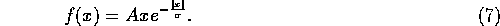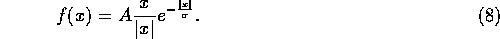Next: The Second Directional Derivative of Image Brightness Up: Edges Previous: Zero Crossings of the Laplacian of a

## More Advanced Uses of the First Image Brightness Derivative

In  Canny described what has since become one of the most widely used edge finding algorithms. The first step taken is the definition of criteria which an edge detector must satisfy, namely, reliability of detection, accuracy of localization and the requirement of one response only per edge. These criteria are then developed quantitatively into a total error cost function. Variational calculus is applied to this cost function to find an ``optimal'' linear operator for convolution with the image. The optimal filter is shown to be a very close approximation to the first derivative of a Gaussian,i.e., in one dimension,Non-maximum suppression in a direction perpendicular to the edge is applied, to retain maxima in the image gradient. Finally, weak edges are removed using thresholding. The thresholding is applied with hysteresis. Edge contours are processed as complete units; two thresholds are defined, and if a contour being tracked has gradient magnitude above the higher threshold then it is still ``allowed'' to be marked as an edge at those parts where the strength falls below this threshold, as long as it does not go below the lower value. This reduces streaking in the output edges.

The Gaussian convolution can be performed quickly because it is separable and can be implemented recursively. However, the hysteresis stage slows the overall algorithm down considerably. While the Canny edge finder gives stable results, edge connectivity at junctions is poor,and corners are rounded, as with the LoG filter.

The scale of the Gaussian determines the amount of noise reduction; the larger the Gaussian the larger the smoothing effect. However, as expected, the larger the scale of the Gaussian, the less accurate is the localization of the edge.

Canny also investigated the synthesis of results found at different scales; in some cases the synthesis improved the final output, and in some cases it was no better than direct superposition of the results from different scales.

Finally, Canny investigated the use of ``directional operators''. Here several masks of different orientation are used with the Gaussian scale larger along the direction parallel to the edge than the scale perpendicular to it. This improves both the localization and the reliability of detection of straight edges; the idea does not work well on edges of high curvature.

In  Deriche uses Canny's criteria to derive a different ``optimal operator''; the difference is that the filter is assumed to have infinite extent. The resulting convolution filter is sharper than the derivative of the Gaussian;This is also implementable as a recursive filter for speed.

In  Shen and Castan describe another related linear operator; the form is,Like the Deriche filter, this is implemented recursively and has an infinite support region. The filter is even sharper than that of Deriche; the argument presented is that the larger the scale of the Gaussian, the more planar the central region, giving rise to an ``unnecessary'' reduction in edge localization. Hence the filter contains a discontinuity at x=0, and information very close to the centre of the filter is given more weighting (and not less, as is usual) than that from slightly further out. However, it is suggested in  that the discontinuity can induce multiple edges. The Shen filter is not separable (in two dimensions), so an approximation to the optimal function must be made.

In practice, the first derivative of the Gaussian, and the Deriche and Shen operators all give very similar results when applied to real images.

In  Monga et. al. extend two dimensional linear filters (in particular, the Shen and Deriche filters) to find edges in three dimensional data such as nuclear magnetic resonance scans.Next: The Second Directional Derivative of Image Brightness Up: Edges Previous: Zero Crossings of the Laplacian of a

© 1997 Stephen M Smith. LaTeX2HTML conversion by Steve Smith (steve@fmrib.ox.ac.uk)